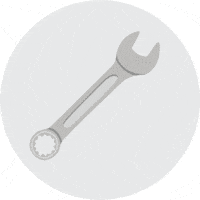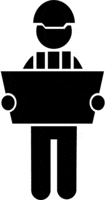# Math Worksheets Land

Math Worksheets For All Ages

# Math Worksheets Land

Math Worksheets For All Ages

Home > Grade Levels > High School Geometry >

# Equations of Circles Worksheets

There are number of manufacturing and design jobs around the world that require companies to create circles that are a perfect circle. They must be the exact needed dimensions and be created by thousands of different machines. How would you share the recipe for making the exact same circle? We can use algebra to write an equation that describe all the key features of that circle. There are number of ways to progress towards writing this equation. We will explore two different methods here. Students can use these worksheets and lessons to learn how to determine the equation of a circle.

### Aligned Standard: High School Geometry - HSG-GPE.A.1

• Answer Keys - These are for all the unlocked materials above.

### Homework Sheets

Given center points and a radius or diameter, tell us more about the circle.

• Homework 1 - Find the equation of a circle whose center is at (3, - 3) and radius 6.
• Homework 2 - The center and the midpoint of the line segment making the diameter AB.
• Homework 3 - Write the equation of a circle whose diameter is 12 and whose center is at (-4, -3)?

### Practice Worksheets

These questions are more diverse than the homework problems.

• Practice 1 - The standard equation of a circle with center C (h, k) and radius r is: (x - h)2+ (y - k)2 = r2
• Practice 2 - The midpoint formula is used to find the coordinates of the center C of the circle.
• Practice 3 - The radius is half the distance between A and B.

### Math Skill Quizzes

You will find the most commonly asked test questions here.

• Quiz 1 - The coordinate of C and the radius are used in the standard equation of the circle to obtain the equation.
• Quiz 2 - Substitute h, k and r in the standard equation to solve
• Quiz 3 - What are the coordinates of the center of this circle? (x + 7)2 + (y + 3)2 = 9.

### How to Find the Equation of CirclesYou must have studied a lot about these shapes? This time around, we are going to focus on how you can find the equations of these glorious figures. First, let's understand the basic definition of this shape. When all the points in a plane are joined together at a fixed distance (known as the radius), from a given point, which is known as the center. In a circle, there are two important features to consider. A line segment passing through the middle of a circle, connecting any two points on the is known as the diameter.

The equation of a circle is a mathematical method to describe the circle. This form of algebra is very helpful when we are working to better understand a coordinate system. The equation represents the position of the circle on a Cartesian plane. There are several different ways in which we can represent the circle using algebra. For the purposes of maintaining our focus on the curriculum for this standard we will examine the general and standard equations of a circle.

The general form is used to specific the specific coordinates of two key features of the circle (the position of the center and the radius). The general form of the equation presents as: x2 + y2 + 2gx + 2fy + c = 0. In this formula the variable g, f, and c are constants.

The standard form of the equation provides you with specific information about all of the same features as the general form and it is often easier to read and understand. This is the method that we would recommend you focus on. With this form we identify the exact position of center with ordered pairs and the position of the point on the circle that forms the radius. Once you know those positions, you just plug them into the formula to produce an equation. The center is identified in this format: (x1, y x1). The arbitrary point on the circumference that helps us identify the radius is presented as (x, y). We simply place these points into the formula: (x - x1)2 + (y - y1)2 = r2.If we are able to determine this formula for this shape, we replicate this figure where and when ever we need it. In addition to being able to replicate this you can use it to model an actual structure. If an engineer can communicate this formula to an architect and the architect to foreman and the foreman to the carpenter, a competent and likely structure that can be reliably built anywhere and everywhere. So remember when you need to do anything with a circle being able to determine the formula will give you powerful information that can change how you view the world.

Unlock all the answers, worksheets, homework, tests and more!
Save Tons of Time! Make My Life Easier Now

## Thanks and Don't Forget To Tell Your Friends!

I would appreciate everyone letting me know if you find any errors. I'm getting a little older these days and my eyes are going. Please contact me, to let me know. I'll fix it ASAP.